Courses

# Laws of Chemical Combinations Class 11 Notes | EduRev

## JEE : Laws of Chemical Combinations Class 11 Notes | EduRev

The document Laws of Chemical Combinations Class 11 Notes | EduRev is a part of the JEE Course Chemistry Class 11.
All you need of JEE at this link: JEE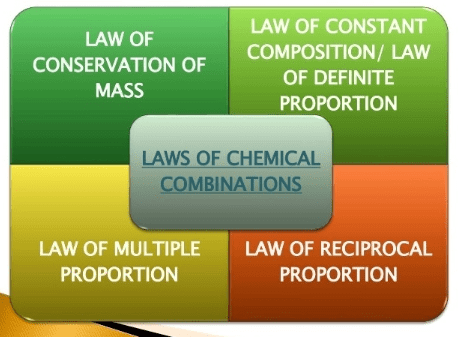In order to understand the composition of the compounds, it is necessary to have a theory which accounts for both qualitative and quantitative observations during chemical changes. Observations of chemical reactions were most significant in the development of a satisfactory theory of the nature of matter. These observations of chemical reactions are summarized in certain statements known as laws of chemical combination.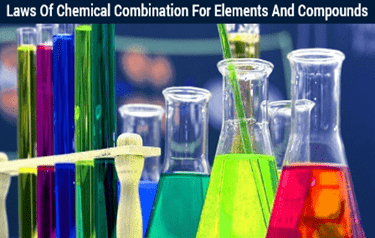1. Law of conservation of mass (Lavoisier): "In a chemical change, total mass remains conserved i.e. mass before the reaction is always equal to mass after the reaction."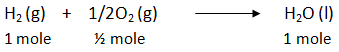mass before the reaction = 1 × 2 + 1/2 × 32 = 18 gm
mass after the reaction = 1 × 18 = 18 gm.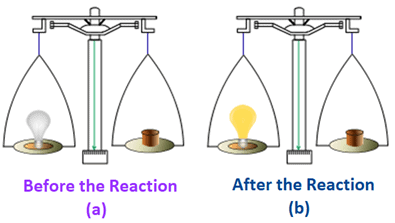Fig: Law of conservation of mass

Example 1. A 15.9 gm sample of sodium carbonate is added to a solution of acetic acid weighing 20.0 gm. The two substances react, releasing carbon dioxide gas to the atmosphere. After the reaction, the contents of the reaction vessel weigh 29.3 gm. What is the mass of carbon dioxide given off during the reaction?
Solution. The total mass of reactants taken = 15.9 + 20.0 = 35.9 gm.
From the conservation of mass, the final mass of the contents of the vessel should also be 35.9 gm. But it is only 29.3 gm. The difference is due to the mass of released carbon dioxide gas. Hence, the mass of carbon dioxide gas released = 35.9 - 29.3 = 6.6 gm.

Example 2. 5.2 g of CaCO3 when heated produced 1.99 g of Carbon dioxide and the residue (CaO) left behind weighs 3.2 g. Show that these results illustrate the law of conservation of mass.
Solution. Weight of CaCO3 taken = 5.2 g
Total weight of the products (CaO +CO2 )= 3.20+ 1.99 = 5.19 g
Difference between the wt. of the reactant and the total wt. of the products
= 5.20 – 5.19 =0.01 g.
This small difference may be due to experimental error.
Thus law of conservation of mass holds good within experimental errors.

2. Law of constant composition (Proust): "All chemical compounds are found to have constant composition irrespective of their method of preparation or sources."
⇒ In H2O, Hydrogen & oxygen combine in 2:1 molar ratio, this ratio remains constant whether it is Tap water, river water or seawater or produced by any chemical reaction.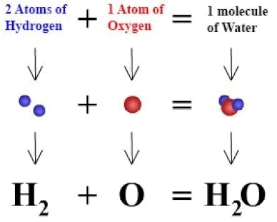Fig: Law of constant composition

Example 1. The following are results of analysis of two samples of the same or two different compounds of phosphorus and chlorine. From these results, decide whether the two samples are from the same or different compounds. Also state the law, which will be obeyed by the given samples.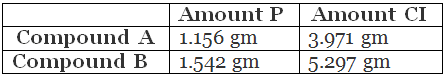Solution. The mass ratio of phosphorus and chlorine in compound A,
mp : mcl = 1.156 : 3.971 = 0.2911 : 1.000
The mass ratio of phosphorus and chlorine in compound B,
mp : mcl = 1.542 : 5.297 = 0.2911 : 1.000
As the mass ratio is the same, both the compounds are same and the samples obey the law of definite proportion.

Example 2. 6.488 g of lead combine directly with 1.002 g of oxygen to form lead peroxide PbO2. Lead peroxide is also produced by heating lead nitrate and it was found that the percentage of oxygen present in lead peroxide is 13.38 percent. Use these data to illustrate the law of constant composition.
Solution.
Step 1: To calculate the percentage of oxygen in first experiment. Weight of peroxide formed
= 6.488 + 1. 002 = 7.490 g.
7. 490 g of lead peroxide contain 1.002 g of oxygen
∴ 100 g of lead peroxide will contain oxygen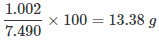i.e. oxygen present = 13.38%

Step 2: To compare the percentage of oxygen in both the experiments.Percentage of oxygen in PbO2 in the first experiment = 13.38
Since the percentage composition of oxygen in both the samples of PbO2 is identical, the above data illustrate the law of constant composition.

Try Yourself!
2.8 g of calcium oxide (CaO) prepared by heating limestone were found to contain 0.8 g of oxygen. When one gram of oxygen was treated with calcium, 3.5 g of calcium oxide were obtained. Show that the results illustrate the law of definite proportions.

3. Law of multiple proportions (Dalton): "When one element combines with the other element to form two or more different compounds, the mass of one element, which combines with a constant mass of the other bear a simple ratio to one another."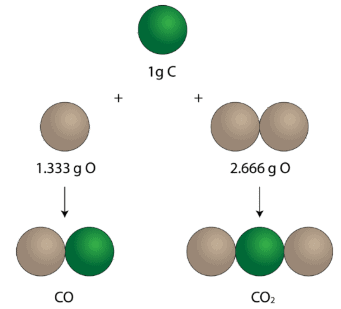Fig: Law of multiple proportions

Carbon is found to form two oxides which contain 42.9% & 27.3% of carbon respectively show that these figures shows the law of multiple proportion.

 First oxide Second oxide Carbon 42.9 27.3 % Oxygen 57.1 % 72.7%

In the first oxide, 57.1 parts by mass of oxygen combines with 42.9 parts of carbon.
1 part of oxygen will combine with 42.9/57.1 = 0.751 part of carbon
Similarly in 2nd oxide,
1 part of oxygen will combine with 27.3/72.7  = 0.376 part of carbon
The ratio of carbon that combine with the same mass of oxygen = 0.751 : 0.376 = 2 : 1
This is a simple whole no. ratio, this means above data shows the law of multiple proportions.

Example 1. Two oxide samples of lead were heated in the current of hydrogen and were reduced to the metallic lead. The following data were obtained
(i) Weight of yellow oxide taken = 3.45 gm; Loss in weight during reduction = 0.24 gm
(ii) Weight of brown oxide taken = 1.227 gm; Loss in weight during reduction = 0.16 gm.
Show that the data illustrates the law of multiple proportion.
Solution. When the oxide of lead is reduced in the current of hydrogen, metallic lead is formed. Definitely, the loss in weight is due to removal of the oxygen present in the oxide, to combine with the hydrogen. Therefore, the composition of the yellow oxide is:
Oxygen = 0.24 gm and lead = 3.45 - 0.24 = 3.21 gm.
The mass ratio of lead and oxygen,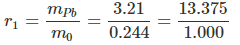and the composition of the brown oxide is : oxygen = 0.16 gm and lead = 1.227 - 0.16 = 1.067 gm.
The mass ratio of lead and oxygen,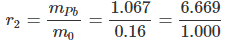Now, r1 : r2 = 13.375 : 6.669 = 2:1 (simple ratio) and hence the data illustrates the law of multiple proportion.

Example 2. Carbon is found to form two oxides, which contain 42.9% and 27.3% of carbon respectively. Show that these figures illustrate the law of multiple proportions.
Solution.
Step 1: To calculate the percentage composition of carbon and oxygen in each of the two oxides
First oxide  Carbon 42.9%   27.3% (Given)
Second oxide Carbon Oxygen 57.1% 72.7% (by difference)

Step 2: To calculate the weights of carbon which combine with a fixed weight i.e., one part by weight of oxygen in each of the two oxides.In the first oxide, 57.1 parts by weight of oxygen combine with carbon = 42.9 parts.
∴ 1 part by weight of oxygen will combine with carbon 42.9/57.1 = 0.751
In the second oxide 72.7 parts by weight of oxygen combine with carbon = 27.3 parts.
∴ 1 part by weight of oxygen will combine with carbon  27.3/72.7 = 0.376

Step 3: To compare the weights of carbon which combine with the same weight of oxygen in both the oxides The ratio of the weights of carbon that combine with the same weight of oxygen (1 part) is 0.751: 0.376 or 2:1

Since this is a simple whole number ratio, so the above data illustrate the law of multiple proportions.

4. Law of reciprocal proportions (Richter): "When two elements combine separately with definite mass of a third element, then the ratio of their masses in which they do so is either the same or some whole number multiple of the ratio in which they combine with each other."
This law can be understood easily with the help of the following examples.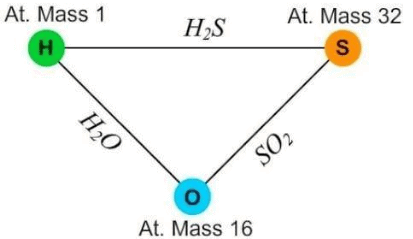Fig: Law of reciprocal proportions

Let us consider three elements: Hydrogen, Sulphur and Oxygen. Hydrogen combines with oxygen to form H2O whereas sulphur combines with it to form SO2. Hydrogen and sulphur can also combine together to form H2S. The formation of these compounds is shown in figure.
Thus, the ratio of masses of H and S which combines with a fixed mass of oxygen is a whole number multiple of the ratio in which H and S combine together.

Example 1. Methane contains 75 % carbon and 25% hydrogen, by mass. Carbon dioxide contains 27.27 % carbon and 72.73% oxygen, by mass. Water contains 11.11 % hydrogen and 88.89% oxygen, by mass. Show that the data illustrates the law of reciprocal proportion.
Solution. Methane and carbon dioxide, both contain carbon and hence, carbon may be considered as the third element. Now, let the fixed mass of carbon = 1 gm. Then, the mass of hydrogen combined with 1 gm carbon in methane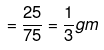and the mass of oxygen combined with 1 gm carbon in carbon dioxide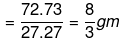Hence, the mass ratio of hydrogen and oxygen combined with the fixed mass of carbon,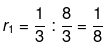Now, the mass ratio of hydrogen and oxygen in water,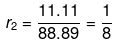As r1 and r2 are same , the data is according to the law of reciprocal proportion.

Example 2. Copper sulphide contains 66.6% Cu, copper oxide contains 79.9% copper and sulphur trioxide contains 40% Sulphur. Show that these data illustrates law of reciprocal proportions.
Solution. In copper sulphides
Cu : S mass ratio is 66.6 : 33.4
In sulphur trioxide
Oxygen : Sulphur (O:S) mass ratio is 60 : 40
Now in CuS
33.4 parts of sulphur combines with Cu = 66.6 parts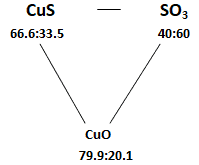40.0 parts of sulphur combines with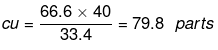Now the ratio of masses of Cu and O which combines with same
Mass (40 parts) of sulphur separately is 79.8:60 …(1)
Cu : O ratio by mass in CuO is 79.9: 20.1 …(2)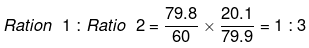Which is simple whole number ratio, hence law of reciprocal proportion is proved.

5. Gay Lussac's law of combining volumes: "When two or more gases react with one another, their volumes bear simple whole number ratio with one another and to the volume of products (if they are also gases) provided all volumes are measured under identical conditions of temperature and pressure."
When gaseous hydrogen and gaseous chlorine react together to form gaseous hydrogen chloride according to the following equation.
It has been observed experimentally that in this reaction, one volume of hydrogen always reacts with one volume of chlorine to form two volumes of gaseous hydrogen chloride. all reactants and products are in gaseous state and their volumes bear a ratio of 1 : 1 : 2. This ratio is a simple whole number ratio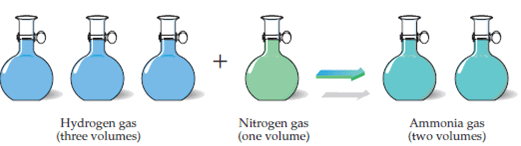Fig: Gay Lussac's Law

Law of combining volumes. These are no longer useful in chemical calculations now but gives an idea of earlier methods of analyzing and relating compounds by mass.

Example 1. 2.5 ml of a gaseous hydrocarbon exactly requires 12.5 ml oxygen for complete combustion and produces 7.5 ml carbon dioxide and 10.0ml water vapour. All the volumes are measured at the same pressure and temperature. Show that the data illustrates Gay Lussac's law of volume combination.
Solution.

Vhydrocarbon : Voxygen : Vcarbon dioxide : Vwater vapour = 2.5 : 12.5 : 7.5 : 10.0
= 1 : 5 : 3 : 4 (simple ratio)
Hence, the data is according to the law of volume combination.

Example 2. How much volume of oxygen will be required for complete combustion of 40 ml of acetylene (C2H2) and how much volume of carbondioxide will be formed? All volumes are measured at NTP.
Solution.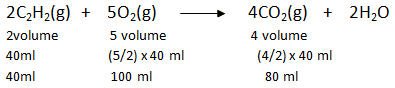So for complete combustion of 40 ml of acetylene, 100 ml of oxygen are required and 80 ml of CO2 is formed.

Try yourself!
Q.1. What mass of silver nitrate will react with 5.85 g of sodium chloride to produce 14.35 g of silver chloride and 8.5 g of sodium nitrate, if the law of conservation of mass is true?
Q.2. Elements X and Y form two different compounds. In the first, 0.324 g of X is combined with 0.471 g of Y. In the second, 0.117 g of X is combined with 0.509 g of Y. Show that these data illustrate the law of multiple proportions
Q.3. 5.06 g of pure cupric oxide (CuO), on complete reduction by heating in a current of hydrogen, gave 4.04 g of metallic copper. 1.3 g of pure metallic copper was completely dissolved in nitric acid and the resultant solution was carefully dried and ignited. 1.63 g CuO were produced in the process. Show that these results illustrate the law of constant proportions.
Q.4. Carbon dioxide contains 27.27% of carbon, carbon disulphide contains 15.79% of carbon and sulphur dioxide contains 50% of sulphur. Are these figures in agreement with the law of reciprocal proportions?

Offer running on EduRev: Apply code STAYHOME200 to get INR 200 off on our premium plan EduRev Infinity!

,

,

,

,

,

,

,

,

,

,

,

,

,

,

,

,

,

,

,

,

,

;